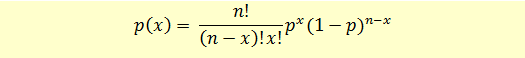# Bernoulli and Binomial Distribution

A Bernoulli random variable is a random variable that takes a value of 1 in case of a success and a value of 0 in case of a failure. We can also say that this random variable has a Bernoulli distribution. A classic example is a single toss of coin. When we toss a coin, the outcome can be heads (success) with a probability p or tails (failure) with a probability of (1 – p). The important point here is a single toss of coin.

Now suppose we perform n number of trials. Each trial is independent and will result in a success with a probability p or a failure with a probability (1-p). From the n trials, suppose X represents the number of successes. Then X is a binomial random variable with parameters (n, p). Note that Bernoulli random variable is a special case of binomial random variable with parameters (1, p). The variable X will have a binomial distribution.

The binomial distribution has the following characteristics:

• For each trial there are only two possible outcomes, success or failure.
• Probability of success, p, of each trial is fixed.
• There are n trials.
• Each trial is independent
• The binomial probability function defines the probability of x successes from n trials.

The binomial probability function is given using the following formula.Let’s take an example to understand how this can be applied.

You have a pool of stocks having returns either above 5% or below 5%. The probability of selecting a stock with above 5% returns is 0.70. You are going to pick up 5 stocks. Assuming binomial distribution, what is the probability of picking 2 stocks with above 5% returns?

Let’s define our problem.